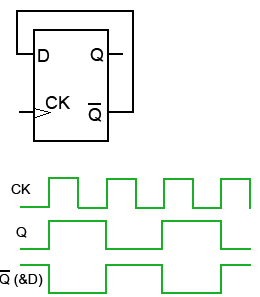Home > sequential logic circuits > toggle flip flop

# Toggle Flip-flop

Toggle flip-flops are the basic components of digital counters, and all of the D type devices are adaptable for such use. When an electronic counter is used for counting, what are actually being counted are pulses appearing at the CK input, which may be either regular pulses derived from an internal clock, or they can be irregular pulses generated by some external event.
When a toggle flip-flop is used as one stage of a counter, its Q output changes to the opposite state, (it toggles) high or low on each clock pulse. Most edge-triggered flip-flops can be used as toggle flip-flops including the D type, which can be converted to a toggle flip-flop with a simple modification. In theory all that is necessary to convert an edge triggered D Type to a T type is to connect the Q’ output directly to the D input as shown in figure. The actual input is now CK. The effect of this mode of operation is also shown in the timing diagram.Toggle Flip-flop Operation
Suppose that initially CK and Q’ = 0. Then Q and D must be 1. At the rising edge of a CK pulse, the logic 1 at D is allowed into the flip-flop and, at the end of the flip-flop’s propagation delay, appears at Q, and Q changes to logic 0 at the same time. This logic 0 is now fed back to D, but it is important that it is not immediately accepted into the D input, otherwise oscillation could occur with D continually changing between 1 and 0. However, because of the flip-flop’s propagation delay, when the logic 0 from Q arrives at D, the very short edge-triggering period will have completed, and the change in data at D will be ignored.
At the next CK rising edge of the clock signal, the 0 at D now passes to Q, making Q and D logic 1 again. The Q output of the flip-flop therefore toggles at each positive going edge of the CK pulse.
Because the Q output changes state at each clock pulse rising edge, the 0 period and the 1 period of the Q output will always be of equal length, and the output will be a square wave with a 1:1 mark to space ratio, its frequency will be half that of CK.